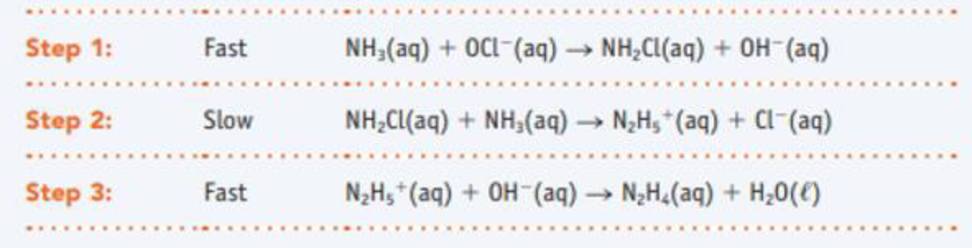Chapter 14.7, Problem 14.13CYU

Chapter
Section
Textbook Problem

The Raschig reaction produces hydrazine, N2H4, an industrially important reducing agent, from NH3 and OCI‒ in basic, aqueous solution. A proposed mechanism is(a) What is the overall equation? (b) Which step of the three is rate determining? (c) Write the rate equation for the rate-determining elementary step. (d) What reaction intermediates are involved?

(a)

Interpretation Introduction

Interpretation: The overall equation has to be given.

Concept introduction:

Molecularity: Elementary steps of a chemical reaction are classified by the number of reactant molecules (or ions, atoms, or free radicals) that come together.  The whole, positive number is called the molecularity of the elementary step.

Rate equations for elementary steps: The rate equation for elementary step is defined by its reaction stoichiometry.

Elementarystep:AProduct.Rateequation:Rate=k[A]

Explanation

The overall reaction is given as,

Overall reaction:The sum of elementary steps:Step1:FastNH3(aq) + OCl-(aq)        NH2Cl(aq) + OH(aq)Step2:SlowNH2Cl(aq) + NH3(aq)    N2H5+ (aq)+ Cl(aq)step 3:  Fast

(b)

Interpretation Introduction

Interpretation: The rate determining step has to be given.

Concept introduction:

The rate of reaction is the quantity of formation of product or the quantity of reactant used per unit time.  The rate of reaction doesn’t depend on the sum of amount of reaction mixture used.

The raise in molar concentration of product of a reaction per unit time or decrease in molarity of reactant per unit time is called rate of reaction and is expressed in units of mol/(L.s) .

The variation in concentration of reaction or product over a certain interval of time is called average reaction rate.

The equation that relates the reaction rate to the reactants concentrations that is raised to various powers is called as rate law.

Rate law can be determined by the slow step or otherwise called as rate-determining step.

(c)

Interpretation Introduction

Interpretation: The rate equation for the rate determining step has to be given.

Concept introduction:

The rate of reaction is the quantity of formation of product or the quantity of reactant used per unit time.  The rate of reaction doesn’t depend on the sum of amount of reaction mixture used.

The raise in molar concentration of product of a reaction per unit time or decrease in molarity of reactant per unit time is called rate of reaction and is expressed in units of mol/(L.s) .

The variation in concentration of reaction or product over a certain interval of time is called average reaction rate.

The equation that relates the reaction rate to the reactants concentrations that is raised to various powers is called as rate law.

Rate law can be determined by the slow step or otherwise called as rate-determining step.

(d)

Interpretation Introduction

Interpretation: The reaction intermediates has to be given.

Still sussing out bartleby?

Check out a sample textbook solution.

See a sample solution

The Solution to Your Study Problems

Bartleby provides explanations to thousands of textbook problems written by our experts, many with advanced degrees!

Get Started

Find more solutions based on key concepts